# Learn what an Excel function is and how it can help you in data analysis

It’s good that you’ve taken an interest in learning what an Excel function is. You’ve probably had formulas in Excel that were too long or too complicated to write manually. So knowing what a function is in Excel will help you to be able to use them instead of formulas. But this is just the beginning!

After you know what is a function in Excel, you will see that it makes a lot of sense to get techniques that will allow you to improve efficiency and productivity in your work. Therefore, we are sure that this guide on what is a function in Excel will add you additional advantages such as:

Having greater agility in the calculation of data.

Reduce unproductive worktime.

what is the structure of an Excel function? what is the difference between a formula and a function? what types of Excel functions are there? Without further ado, we are ready to answer the above questions and embark on the journey that will allow you to save time when analyzing data in Excel spreadsheets.

let’s get started!

## what is a function in Excel?

An Excel function is a predefined operation that performs calculations using specific values (arguments) in a particular order (structure). they are mainly created to save us time and to let Excel take care of everything else.

“In other words, Excel takes care of the entire calculation, formula or action for us, and only asks us to specify the function and data; as the arguments,” explains Julieta Santarelli, a public accountant with a master’s degree in Management & Analytics, who teaches the basic Excel course for beginners.

In practical terms, to understand what a function is in Excel, we can see it as a kind of shortcut codes that save us from having to write a whole mathematical operation or a whole calculation/analysis of certain data.

## what is the structure of a function in Excel?

Now, after understanding what is a function in Excel, you must learn how to apply a function in Excel, for which it is necessary that you know what is the syntax or structure of a function in Excel.

In this regard, Julieta Santarelli indicates the parts of a function in Excel, understanding that a function is always composed of the following elements:

Equal sign (=) + the name of the formula: to tell Excel to perform the calculation and return the result of the formula that we will write next.

An opening parenthesis: inside which we are going to begin to introduce the arguments of the function, for that you must have clear what is the argument of a function in Excel, as we explain in the following element.

Arguments: they can be a constant (word, number, date), a reference (cell or range) or a function (which would be nested functions). These must be separated by commas (,) or semicolons (;), depending on the configuration.

Finally, a closing parenthesis: when we press enter, we will have the result.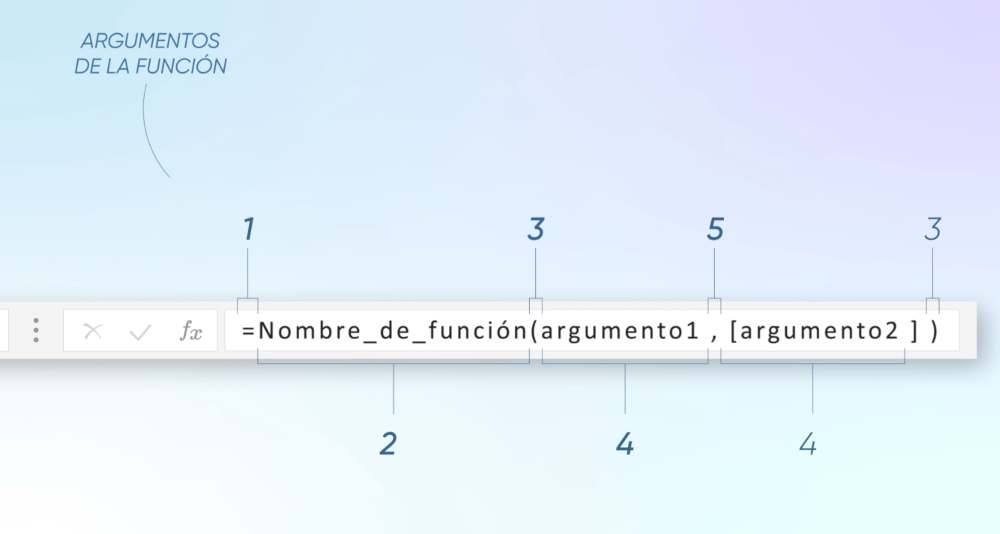Source: GG

As you can see, each function in Excel has its own syntax, which you must strictly respect. You may be thinking, but how hard is Excel! On the one hand, maybe you are right. All you have to do is make a mistake by putting an extra comma (,) and you will have to repeat the functions in Excel from the beginning.

Actually, Excel comes to our benefit if you know how to handle it well. In fact, there is a function called Excel Function Wizard that helps us to write them correctly, greatly reducing the chances of making a mistake.

Now that we understand what a function in Excel is and what its syntax is, you should also know how to differentiate it from another term that is very commonly used, but closely linked to the function in Excel: the formula.Excel workflow template for planning your projects

what is the difference between a formula and a function in Excel?

To better identify what is a function in Excel, it is necessary to differentiate what is a formula and what is a function in Excel.

### Formulas in Excel

On the one hand, a mathematical formula is “a set of operations between numbers and mathematical operators, which solve a calculation by returning a result”. In this sense, if we have numerical data, we can make several formulas in the Excel template, just as if we were using a calculator with mathematical operators, which we already know: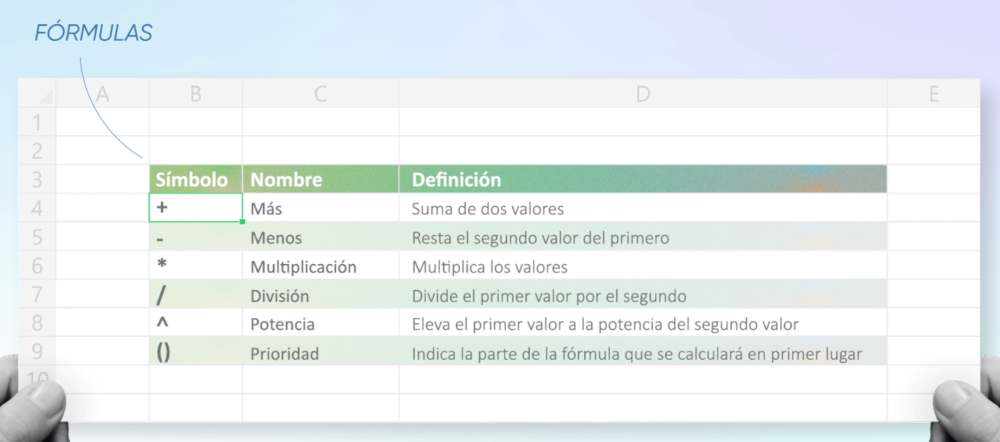Source: GG

According to Microsoft Support, the formula in Excel “always starts with an equal sign (=), followed by numbers, mathematical operators (such as plus and minus signs) and functions, which can extend the power of a formula”,

Example of a formula in Excel can be the multiplication of 4 by 8: =4*8.

When typing it in an Excel cell and pressing enter, the system has to return the result: 32. In the same way, it can be done with cell coordinates that contain values, as we will see in the following image, where 4*10 (B5*C5) will be multiplied, and 6+6+10 (B7+CY+D7) will be added: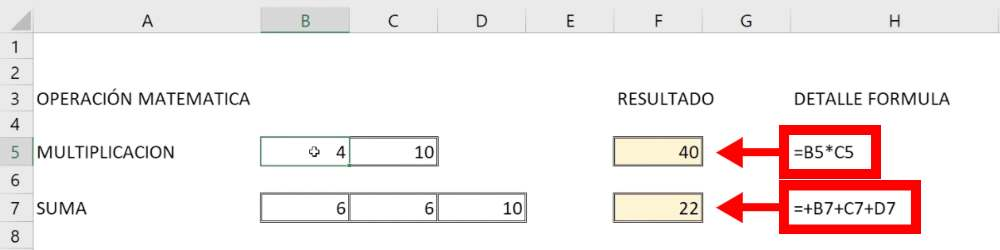Source: GG

### Functions in Excel

On the other hand, examples of function in Excel are: SUM, SEARCH, EXTRAE, etc., which function as a kind of ‘shortcodes’ that can be used to execute simple or complex operations .

In the following example we will repeatedly add the number 2, but in this case we will not need to put the plus sign (+) after each data to add, but we will use the SUM function: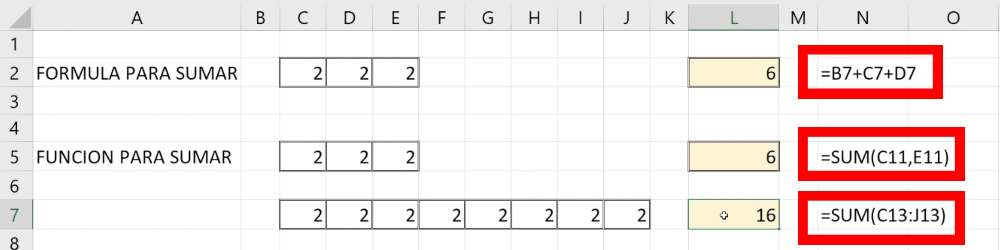Source: GG

## what types of Excel functions are there?

And if you’re wondering what Excel functions are, you should know that there are different groups and that they are categorized, redundantly, according to their function.

Excel has a large number of functions that allow you to do anything you can think of. Moreover, you can combine different functions, as is the case in some advanced Excel formulas.

### Mathematical and trigonometric functions

A mathematical function in Excel is used to do mathematical and trigonometric calculations, using numerical data. For example: function =SUM(), or function =PRODUCT().

### Statistical functions

They allow to calculate statistical analysis of data, such as maximum =MAX(); minimum =MIN() and averages =AVERAGE(). There are also the function =COUNT() and the function =COUNT.IF().

### Logical functions

They allow you to evaluate logical expressions and decide the course of action based on their result. Examples of this group are the functions =SI(), =Y() or =O().

### Date and time functions

As their name indicates, they allow us to perform operations with this type of data, such as function =Time(), function =DATE() and function =DATE().

### Text functions

They allow us to transform text data or to construct new text data, such as the function =CONCATENATE().

### Search and reference functions

We use them to find values or references in our spreadsheets in our search sheets. The best known of this group is the function SEARCHV().

### Information functions

They are used to find out types of data stored in cells and obtain specific information about them. One of them is the function =WRONG().

### Other groups of Excel functions

The use of a function in Excel within a formula is in some cases necessary. Sometimes it is not possible to perform complicated calculations with only mathematical functions. An example is the use of the TAN function, which allows you to calculate the tangent of an angle. So other groups of Excel functions are:

Compatibility functions

Cube functions

Database functions

Engineering functions

Financial functions

Web functions

## how to create basic functions in Excel?

congratulations, we are very happy that you are still learning with us. You already know what a function in Excel is, the difference between a formula and a function, the parts of a function in Excel and the types of functions there are. So now it’s time to learn how to make basic functions in Excel.

First of all, the main tool for using functions in Excel are cells, the fundamental unit of Excel. It is in the cells where you are going to write the logical expressions, the arguments and it is also the place where the results are displayed. In this case, we will choose C6 to give the result.
To enter a formula, you can type directly in the cell, or use the function bar, the table above the calculation table (you identify it by the symbol fx). The first point to notice is the equals sign (=), which is not part of the formula itself, but serves to inform the tool that you are entering a function in Excel and that you are asked to calculate the arguments entered after the symbol. In this case, we will write =SUM(C2:C5) without spaces.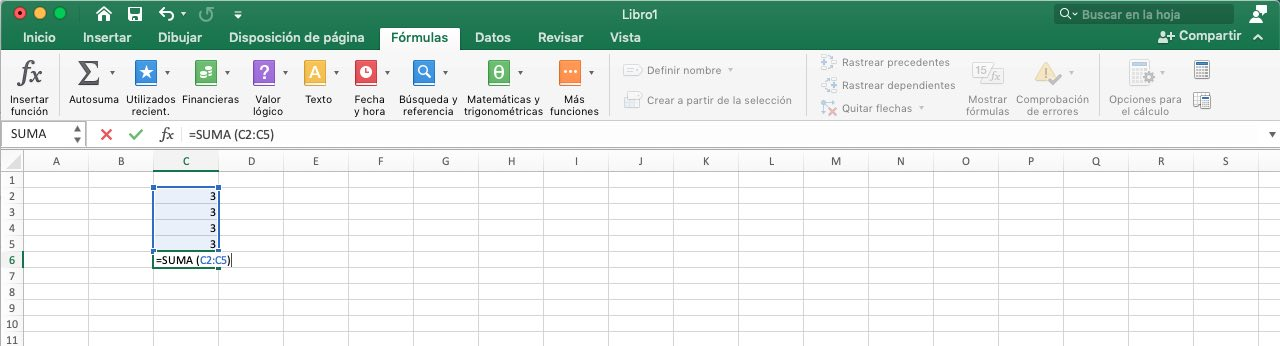Image: Hans’ computer screenshot

Taking the example of the Excel function sum (), the addends are placed in parentheses, while the result is displayed directly in the cell. As you can see in the following image, once you send the calculation, cell C6 shows the value 12, the total, instead of the sum of 3 plus 3 plus 3 plus 3 that was entered at the beginning. Actually, the formula has not disappeared. In fact, if you select the cell, you can see the formula in the fx bar, and if you double-click on the cell, you will even see that you can modify it.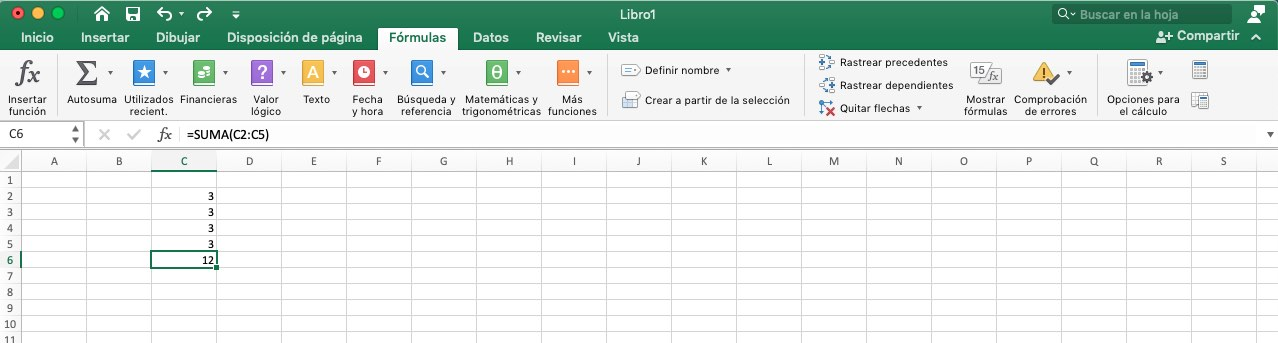Image: Hans’ computer capture

By the way, today we want to share with you a sales forecast template in Excel that will be useful to optimize the business processes within your organization.

### Excel Function Argument

We have already explained what a function argument in Excel is. Then, when you read the Excel function example above, you must have noticed that all Excel functions use parentheses. The information used by the functions and contained inside the parentheses, separated by semicolons, are the arguments. Each function uses different arguments. Therefore, an Excel function can go:

No arguments

One argument

A fixed number of arguments

An indefinite number of arguments

Optional arguments

An example of a function in Excel that does not use any arguments is the function NOW, This Excel function displays the current date and time. Even if a function does not use an argument, you need to use empty parentheses, like this:

=DATE()

If you use a function in Excel that uses more than one argument, each argument must be separated with a semicolon. An argument can be a cell reference, a numeric value, a set of text, a mathematical expression and even other functions (nested functions). Here are some examples of Excel functions that use various types of arguments:

Cell reference: = SUM(C1:C24)

Text string: = = = SHIFT.INIZ(“hans baumann”)

### The syntax of the functions

Therefore, the standard structure of a function in Excel is as follows:

=FUNCTION NAME(Argument 1; Argument 2; etc.).

Pro tip: remember that there is never a space between the function name and the parenthesis you have opened. It is possible to insert a space after the comma between the arguments in parentheses (if you wish), but this is only a matter of readability and does not affect functions in Excel. The arguments of a function must be entered in the correct order.

## how to access the most common Excel functions more quickly?

All Excel functions are available in the command group of the Function Library on the FORMULAS tab.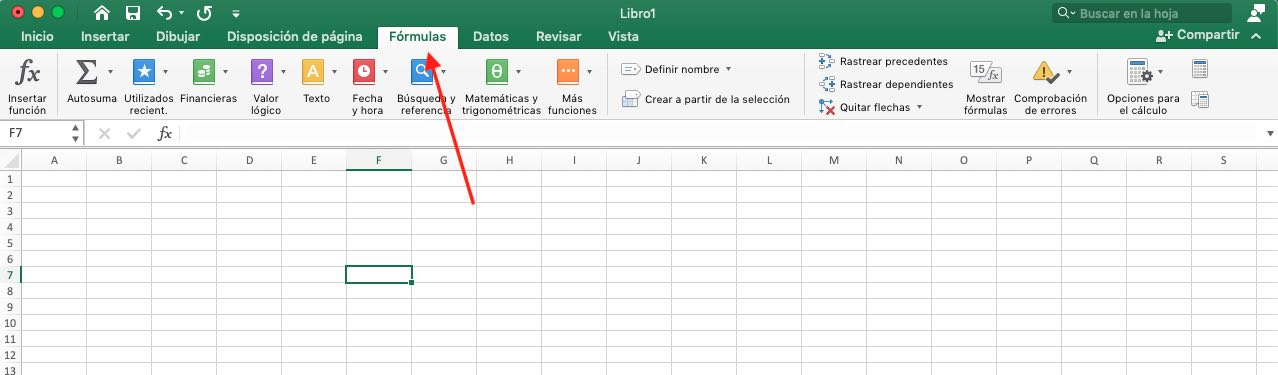Image: Hans’ computer capture

Excel functions are divided by categories (Financial, Logical Value, Text, etc.). Clicking on each of the categories displays a list. Now, how to access the most common Excel functions more quickly?

It is very easy through the AutoSum command, which allows you to select the most common Excel functions (Sum, Average, Count numbers, Max, Min, etc.), or through the More functions option, you can access any Excel function. The tool also allows you to access the complete list of its functions by clicking on the Insert function button.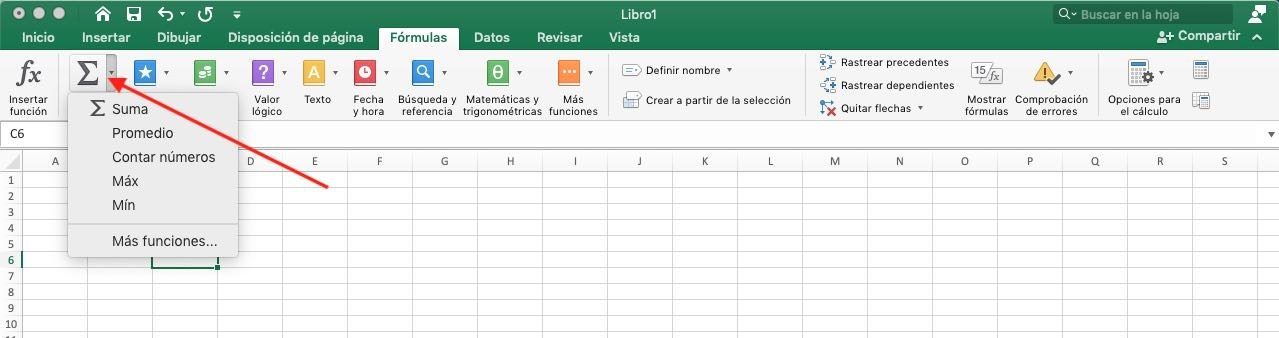Image: Hans’ computer capture

To use a function in Excel in a more agile way, you can use the Insert function button on the left of the formula bar.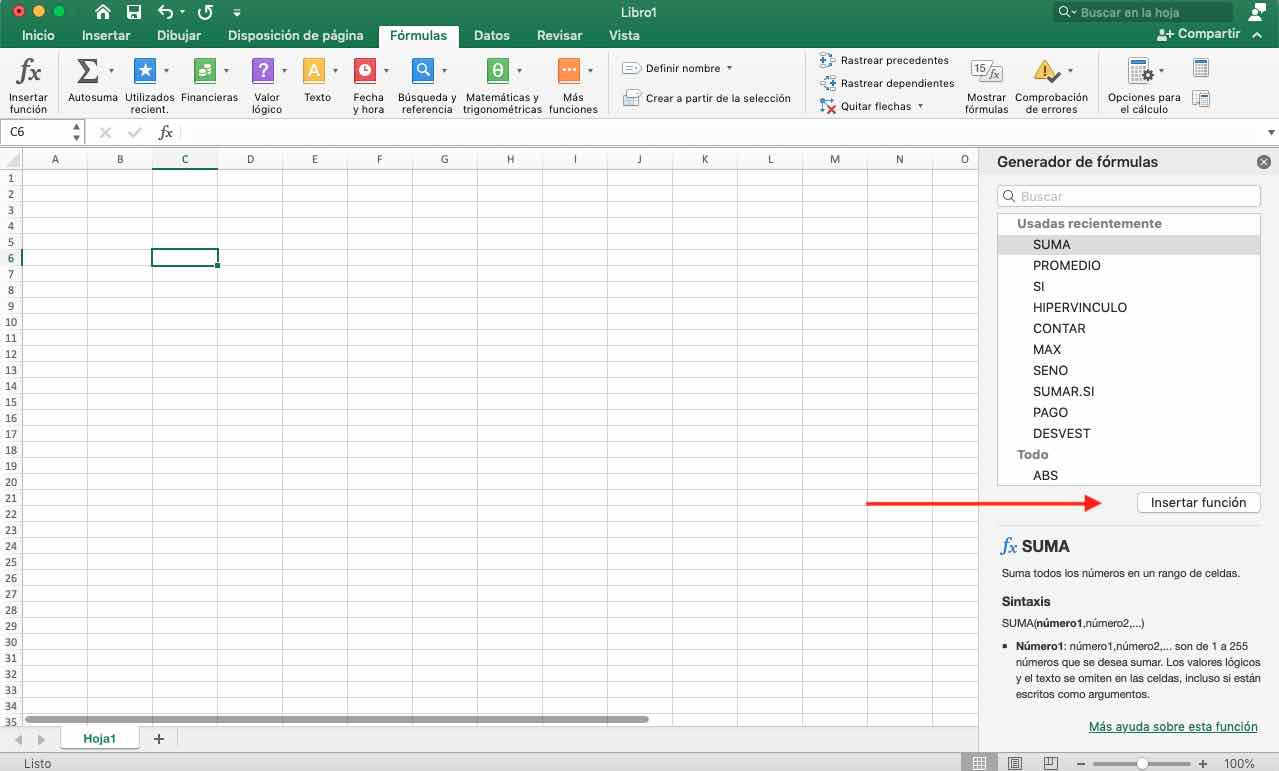Image: Hans’ computer screenshot

Once you have selected the function in Excel, a dialog box opens in which you have to enter the arguments. Obviously, as mentioned above, the arguments will be different depending on the function you choose. In the following image we see, for example, the arguments required by the Count Numbers command.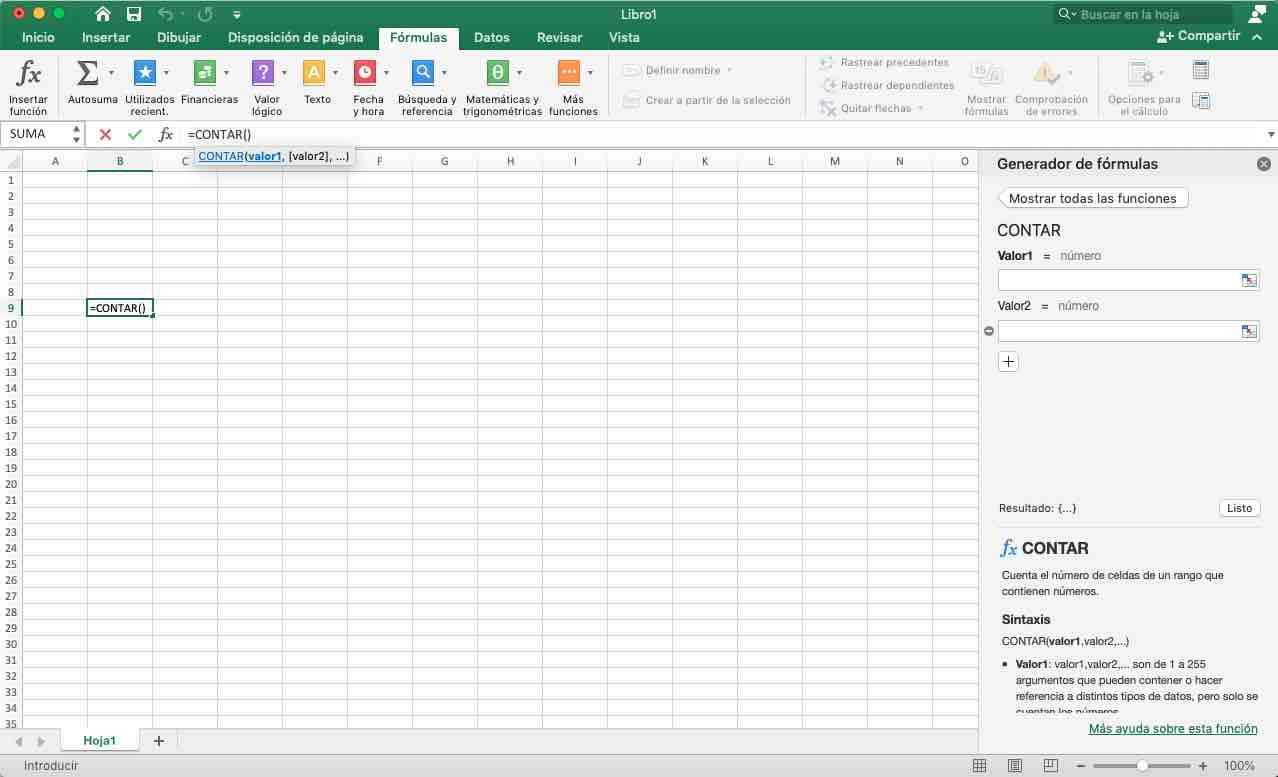Image: Image: computer capture from Hans

After you have entered the required arguments, just click the Done button, and Excel will do the rest. Of course, in addition to inserting the function, it is also possible to type it manually into the cell of your choice. And that’s it!now that you know what a function in Excel is and what it is used for, you can start laying the foundations of your accounting projects with a full understanding of the workings and nature of Excel.

As you have noticed, Excel’s built-in functions are sort of like “microprograms” that can be inserted into formulas, either to simplify them or to perform complex calculations.

see you next time!